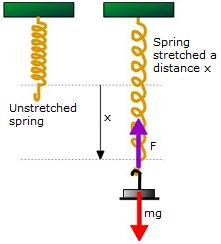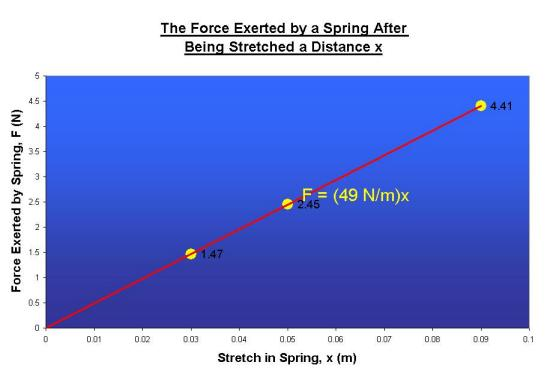### One way to measure a spring constant.

Watch The Video

Teachable Topics:

• Hookes Law
• Springs
• Elasticity

Theory:

Physicists seem to pay a lot of attention to springs. But why!??! Aside from the spring on the verandah door, the one on the pogo stick that's been lying in the garage since 1962, and the springs in the shock absorbers on your car, they don't seem to play a big part in the world around us. However, physicists recognize that springs are useful models for other phenomena. Part of the reason that a spring makes a good model for other phenomena is that it's simple. The force law for a spring looks like this: F = - kx where F is the force exerted by a spring, x is the displacement of the end of the spring from its equilibrium position, and k is what is called the spring constant. The spring constant is a measure of the "stiffness" or "strength" of a spring. To determine this spring constant, you could do the following: hang the spring from some sort of support and note its unstretched length. Now add some mass to one end of the spring. The spring will stretch and come into equilibrium at a length x beyond the spring's unstretched length. The masses are pulled down by force of gravity and pulled up by the spring force. The two forces balance, and this means that the force of the spring on the masses is equal to mg, the weight of the masses. Adding more mass will further stretch the spring. By measuring and plotting the spring force, F, againt the stretching of the spring, x, you should get a straight-line graph with slopeFigure 1

Apparatus:

• Meter stick
• Various weights
• Springs

Procedure:

• Suspend a spring from a support, and align the zero point of a measuring stick with the bottom end of the spring.
• Hang a hanger from the end of the spring and load it with a weight.
• Record the mass of the hanger and weight along with the stretch of the spring.
• Add more weights to the hanger and again measure the mass and stretch of the spring.
• Repeat this several times, then use the measured masses to calculate the spring force at each step in the experiment.
• Plot the spring force against the stretch in the spring - the graph should be a straight line whose slope is equal to k, the spring constant for the particular spring you're using.

Below is the graph that uses the data from the experiment in the video. Its slope was calculated to be 49 N/m.